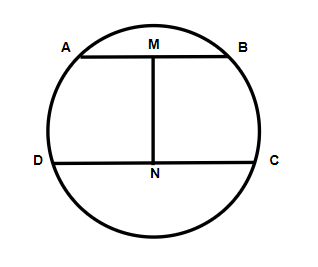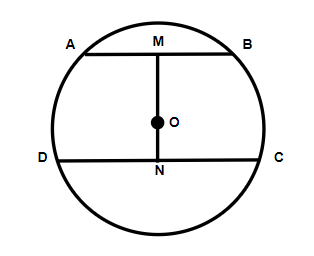In the given figure, chords $AB$ and $CD$ are parallel. $M$ and $N$ are midpoints of $AB$ and $CD$ respectively. Choose the correct statement or statements.$I$ . $MN$ is definitely perpendicular to both $AB$ and $CD$ .$II$ . $MN$ will definitely pass through the center of the circle.$\left( a \right){\text{ Only I is correct}}$$\left( b \right){\text{ Only II is correct}}$$\left( c \right){\text{ Both are correct}}$$\left( d \right){\text{ Both are incorrect}}$Verified
120.6k+ views
Hint: This type of question is solved by using the statement so if we prove that $AB$ and $CD$ are parallel then we will be able to validate the statements given in this question. As we realize that the line bisecting the chord and going through the focal point of the circle is opposite to the chord. So by using all this information, we can easily come to the point to choose the option.

Since in the question it is given that chords $AB$ and $CD$ are parallel.
So, we realize that the line bisecting the chord and going through the focal point of the circle is opposite to the chord.
First of all, we will assume $O$ be the point which will be the center of the circle. So in the figure, it will look like,Hence, the angle $\angle OMA = \angle OND = {90^ \circ }$ each because of the alternate interior angles.
Hence, from this, we can say that $MN$ will definitely pass through the center of the circle.
As we can see here the line is passing through the center of the chords. So the chord will be bisected equally into two parts.
Hence, we can say that
$\Rightarrow AM = MB$
And similarly, for the chord $CD$ , we have
$\Rightarrow CN = ND$
Therefore, from this, we can say that $ON$ will be perpendicular to $CD$ and also similarly $OM$ will be perpendicular to $AB$ .
Hence from this, we can say that $MN$ is definitely perpendicular to both $AB$ and $CD$ .
Hence, the option $\left( c \right)$ is correct.

Note: A chord will be the line fragment and it joins two focuses on the boundary of a circle, and the chord which will be the longest of a circle is the width having the diameter. Since the breadth goes through and contains the focal point of the circle.